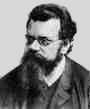Home

Lecture Notes

PHY 418: Statistical Mechanics I
Prof. S. Teitel stte@pas.rochester.edu ---- Spring 2011

## Lecture Notes

The lecture note files correspond roughly to the material presented in a given day's lecture. But you may on occassion find the end of one day's lecture at the start of the file for the next day's lecture, so please look there if you think there might be something missing.

• Lecture 0 - Sketching the history of statistical mechanics and thermodynamics (another such page here.)

• Lecture 1 - Postulates of classical thermodynamics, entropy, temperature, pressure, chemical potential

• Lecture 2 - Conditions for thermal, mechanical, and chemical equilibrium, concavity of the entropy, equations of state, Euler relation, Gibbs-Duhem relation

• Lecture 3 - Entropy of the ideal gas, entropy maximum vs energy minimum principle

• Lecture 4 - Legendre transformations, Helmholtz and Gibbs free energies, enthalpy, the grand potential, reservoirs, extremum principles for these new thermodynamic potentials

• Lecture 5 - Maxwell Relations, response functions and relations between them, curvature of free energies and stability requirements for response functions

Lecture 5 supplement - Heat engines, thermodynamic efficiency, Carnot cycle
• Lecture 6 - Kinetic theory of ideal gas, ergodic hypothesis, statisitcal ensembles, density matrix, Liouville's theorem

• Lecture 7 - Microcanonical ensemble, density of states and connection to entropy, entropy of the ideal gas and problem of extensivity

• Lecture 8 - Entropy of mixing and Gibbs' paradox, indistinguishable particles, the canonical ensemble

• Lecture 9 - Helholtz free energy and the canonical partition function, energy fluctuations, equivalence of canonical and microcanonical ensembles in the thermodynamic limit, average energy vs most probably energy, Stirling's formula

• Lecture 10 - Factoring the canonical partition function for non-interacting objects, Maxwell velocity distribution revisited, the virial theorm

• Lecture 11 - The equipartition theorem, elastic vibrations of a solid and the law of Dulong and Petit, paramagnetism of classical spins and the Curie law of paramagnetic susceptibility

• Lecture 12 - Entropy and information

• Lecture 13 - The grand canonical ensemble: the grand canonical partition function and the grand potential, fluctuations in the number of particles

• Lecture 14 - Fluctuations in the grand canonical ensemble, the grand canonical partition function for non-interacting particles, chemical equilibrium, a gas in equilibrium with a surface of absorption sites

• Lecture 15 - Quantum ensembles, density matrix, quantum microcanonical, canonical, and grand canonical ensembles

• Lecture 16 - Quantum harmonic osciallator at temperature T, Bose-Einstein and Fermi-Dirac statistics, Pauli exlusion principle, occupation number representation, density matrix for two non-interacting particles

• Lecture 17 - Quantum correlations as an effective classical pair interaction, N-particle partition function in the position-space basis, partition functions for non-interacting quantum ideal gas, classical partition function in the occupation number representation

• Lecture 18 - Average occupation number, the classical vs quantum limits of the ideal gas, the quantized harmonic oscillator as bosons

• Lecture 19 - Debye model for the specific heat of a solid, black body radiation

• Lecture 20 - The quantum ideal gas, standard functions, pressure, density, energy, the leading correction to the classical limit, the degenerate Fermi gas and the Fermi energy

• Lecture 21 - The degenerate Fermi gas, ground state energy, the Sommerfeld expansion, chemical potential at finite temperature, specific heat

• Lecture 22 - Pauli paramagnetism of a degenerate electron gas

• Lecture 23 - Bose-Einstein condensation in an ideal gas of bosons

• Lecture 24 - BEC in laser cooled atomic gases, classical spin models, constant magnetization vs constant magnetic field ensembles

• Lecture 25 - Broken symmetry and the possibility for a phase transition in the thermodynamic limit, Ising phase diagram

• Lecture 26 - Mean-field solution of the Ising model, critical exponents

• Lecture 27 - Mean-field solution and critical exponents continued, Maxwell construction, Landau theory of phase transitions

• Lecture 28 - Landau theory continued, critical exponents, specific heat, exact solution of one dimensional Ising model

• Lecture 29 - Fluctuations and diverging length scale at a 2nd order critical point, Landau-Ginzburg theory, correlation functions, upper and lower critical dimensions (this lecture was not presented in class -- I post it here for those of you who are interested)